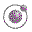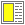svg-paths.scm - A simple Scheme path library developed for SVG. Lecture 3 - slide 31 : 42Program 3

```;;; Scheme SVG path functions.
;;; Functions for definition of SVG paths

;; Move start of path to x y - absolute
(define (am-p x y path)
(string-append "M " (as-string x) " " (as-string y) " " path))

;; The emtpy path
(define (e-p) "")

; Absolute line path to x,y
(define (al-p x y path) (p-exp "L" path x y))

; Relative line path to x,y
(define (rl-p x y path) (p-exp "l" path x y))

; Absolute horizontal path
(define (ah-p x path) (p-exp "H" path x))

; Relative horizontal path
(define (rh-p x path) (p-exp "h" path x))

; Absolute vertical path
(define (av-p y path) (p-exp "V" path y))

; Relative vertical path
(define (rv-p y path) (p-exp "v" path y))

; Relative move to - without drawing
(define (rm-p x y path) (p-exp "m" path x y))

; Absolute move to - without drawing
(define (am-p x y path) (p-exp "M" path x y))

; Closed path
(define (z-p) "Z")

; SVG render path
(define (p-exp letter path . coordinates)
(string-append
letter " "
(list-to-string (map as-string coordinates) " ")
" " path))

(define concat-p string-append)

(define (box . four-numbers)
(list-to-string (map as-string four-numbers) " "))```